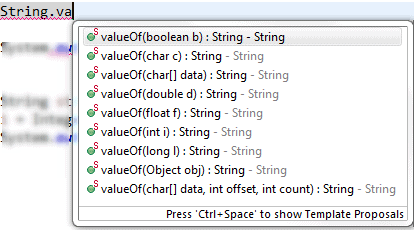JavaForQAAbove screenshot gives you size of each data type in java, which will give us an idea of compatibility of data type conversion and the result if you forcefully convert one to another type.

String to int

```String str = "234";
int i = Integer.parseInt(str);```

int to double

```int i = 10;
double d = i;
System.out.println(d);```

Output : 10.0

double to int

```double d1 = 13.56;
int i2 = (int) d1;
System.out.println(i2);```

Output : 13

long to int

```long l = 2147483647L;
int i1 = (int) l;
System.out.println(i1);```

Output : 2147483647

here the value of long variable is with in the range of int, that’s why no loss in data while conversion, but if we keep long value more than int range, there will be data loss, let’s take a look at it –

```long l = 2147483647123L;
int no = (int) l;
System.out.println(no);```

Output : -877

same is with byte and short data type while converting long to byte or short type.

Other datatype to StringvalueOf(<datatype> <variable>) method allows us to convert any datatype like int, char, long, Object etc to String

[as shown in the above screenshot]

```int i = 980;
String str = String.valueOf(i);
System.out.println(str);```

output : 980

Another method is to use toString(<primitive dataType> <variable>), example as follows

```int i = 100;
String str = Integer.toString(i);
System.out.println(str);```

output : 100

toString() method basically uses respective Object types (Integer, Byte, Long etc) to convert primitive types(int, byte, long etc) to string.